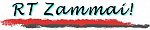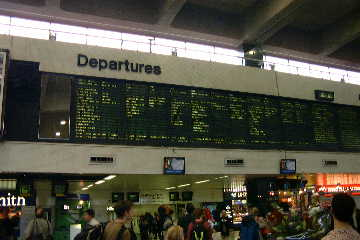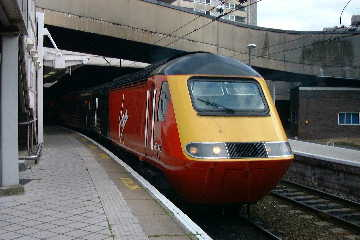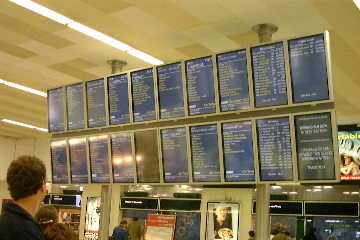Sitemap | Coverstory | Photos | Railways z. | Bridges z. | Seeds
\$B8=:_\$N%Z!<%8(B :
 \$B%H%C%W(B >> \$B%P!<%_%s%,%`JX\$j(B >> 2001\$BG/(B >> 7-9\$B7n(B >> \$B%G%8%+%aJX\$j(B \$BBh(B2\$B2s(B
You are now in :
 Top >> From Birmingham >> Yr. 2001 >> 3rd Q. >> Digital Camera Report No. 2

\$B%P!<%_%s%,%`!&%G%8%+%aJX\$j(B
Digital Camera Report from Birmingham

# \$BBh(B2\$B2s(B \$B!V%O%C%H%U%#!<%k%I0J8e!W(BNo. 2: "After Hatfield"

 When I arrived at London Euston terminal on Sunday 1 July, there were many people looking up the Departure signs. Yes, trains were late, as usual... Almost a year after the train crash at Hatfield in October 2000, which caused so much trouble throughout the British rail network, the confusion was nearing its end, although the weekend services were still likely to delay. It seemed to me that passengers, government and rail operators are all coming to a point where they cannot withstand the chaos any more. It seems apparent that there was a flaw in the framework of the reorganised rail industry in itself. The train I took from Euston on the day seemed to be HST, a diesel loco hauled train that can divert to Nuneaton and run through non-electrified section between Nuneaton and Birmingham.

## \$B\$"\$"!"(BEuston \$B\$N?M:.\$_(B

\$B1Q9qF~\$j\$O!"(B \$B8=CO;~4V(B6\$B7n(B30\$BF|EZMKF|AaD+!"(B \$B%m%s%I%s!&(BHeathrow \$B6u9ACe!#(B \$BBg3X\$H\$N7@Ls3+;OF|\$N(B7\$B7n(B1\$BF|\$OF|MKF|\$@\$+\$i!"(B 30\$BF|!&(B1\$BF|\$NN>F|\$G;~:9\$\\$1\$rD>\$7!"(B \$B1Q8l\$K<*\$r47\$i\$9\$3\$H\$,\$G\$-\$=\$&\$@!#(B \$BEZMKF|\$O%m%s%I%s\$GB?>/\$N4Q8w!#(B \$BF|MKF|\$N8aA0Cf\$K\$5\$C\$5\$H%m%s%I%s\$rH/\$F\$P!"(B \$B8a8e\$K\$O\$3\$l\$+\$iJk\$i\$939\$N\$h\$&\$9\$rB?>/\$O8+\$F2s\$l\$k\$K0c\$\$\$J\$\$\$H;W\$C\$?!#(B \$B%m%s%I%s\$+\$i%P!<%_%s%,%`\$^\$G\$OLs(B180km\$BDxEY!"(B \$BNs \$B\$H\$\$\$&\$o\$1\$G!"(B 7\$B7n(B1\$BF|\$ND+(B10\$B;~\$3\$m!"(B \$BKM\$O%m%s%I%s!&(BEuston \$B!J%f!<%9%H%s!K(B \$B%?!<%_%J%k\$G!"(B \$BNse\$2\$k0l72\$N?M!9\$N\$J\$+\$K\$\$\$?!#(B

\$B\$d\$i\$l\$?\$J!"(B \$B\$H;W\$C\$?\$b\$N\$@!#(B

\$B9M\$(\$F\$_\$k\$H!"(B \$B%m%s%I%sCO2h\$k\$N\$O(B1995\$BG/0JMh\$@!#(B \$BEv;~Ns \$B:Y\$+\$\$;z\$G\$\$\$m\$s\$J\$3\$H\$,=q\$\$\$F\$"\$kI=<(4o\$rI,;`\$KFI\$s\$G!"(B \$B2?\$H\$+L\E*\$NNs!)(B   \$B\$=\$&\$+!"(B \$B\$3\$l\$r\$_\$s\$JBT\$C\$F\$\$\$k\$N\$@!"(B \$B\$HG[Photo-1] London Euston \$B1X\$N=PH/0FFbI=<(!#1U>=I=<(\$i\$7\$\$\$,8+\$K\$/\$\$\$3\$H\$3\$N>e\$J\$\$!#(B \$B!J(B2001. 9. 21 \$B;#1F!#(B\$BBg\$-\$J2hA|(B(JPEG): 93kB\$B!K(B Departure signs at London Euston terminal. It seems to be liquid crystal display, but is very hard to see. (Photo taken: 21 Sep 2001. Larger image (JPEG): 93kB)

## \$B2fK}\$N8B3&(B

\$B;v8N\$+\$i(B1\$BG/6a\$/\$r7P\$?8=:_!"(B \$B%P!<%_%s%,%`\$^\$o\$j\$NE4F;\$OJ?F|\$J\$iHf3SE*\$^\$H\$b\$KAv\$k\$h\$&\$K\$J\$C\$?\$h\$&\$@!#(B \$B\$7\$+\$7!"(B \$B=5Kv\$N%@%\$%d\$NMp\$l\$OAjJQ\$o\$i\$:\$@!#(B \$BCO6h\$K\$h\$C\$F\$O!"(B \$BJ?F|\$K\$bAjEv\$JMp\$l\$,;D\$k\$H\$3\$m\$b\$^\$@\$"\$k!#(B

\$B\$3\$A\$i\$KMh\$F\$+\$i\$^\$@Bg\$7\$F;~4V\$,\$J\$\$\$,!"(B \$B\$3\$N>u67\$K4X\$9\$kJsF;\$K\$O(B \$B!JJF9q\$N%F%m;v7o\$NA0\$K\$OFC\$K!K(B \$BIQHK\$K@\\$9\$k\$3\$H\$K\$J\$C\$?!#(B

\$B>h5R\$b!"(B \$B@/I\\$b!"(B \$B\$"\$k\$\$\$O\$*\$=\$i\$/1?1D\$K7H\$o\$k4X78 BBC \$B\$NJsF;\$K\$h\$l\$P!"(B \$B>h5R\$NN)>l\$+\$iE4F;;:6H\$r4F;k\$9\$k8xE*5!4X(B Rail Passengers Council (RPC) \$B\$O!"(B \$B\$3\$N>u67\$r(B \$B!V@o;~Cf\$r=|\$1\$PE4F;;K>e:G0-\$NM"Aw:.Mp!W(B \$B\$H;XCF\$7\$?!#(B \$B\$3\$N>u67\$O:#8e\$b\$9\$0\$K2~A1\$9\$k8+9~\$_\$O\$J\$\$!#(B Railtrack \$B\$N?7u67\$O\$"\$H(B4\$BG/DxEYB3\$/\$H\$\$\$&\$7!"(B RPC \$B\$K\$h\$l\$PC;4|E*\$K\$OE_5(\$K:F\$S>u67\$,0-2=\$9\$k2DG=@-\$5\$(\$"\$k\$H\$\$\$&!#(B

Hatfield \$B;v8N8e\$N:.Mp\$b\$"\$C\$F!"(B \$BE4F;%M%C%H%o!<%/\$N3HD%\$d2~A1\$K\$+\$+\$o\$k7W2h\$NB?\$/\$,0l;~Dd;_\$rM>57\$J\$/\$5\$l\$F\$\$\$k!#(B \$B\$3\$l\$i\$N7W2h\$O!"(B \$BNse\$7!"(B Edinburgh \$B\$K;j\$k!K(B \$B\$N(B2003\$BG/0J9_\$N%U%i%s%A%c%\$%:\$K\$D\$\$\$F!"(B \$B8=:_\$N1?9T2qF3F~\$N\$?\$a\$NEj;q\$r1?9T2q \$B;v8NA0\$+\$i0-\$+\$C\$?E4F;\$NI>H=\$O!"(B \$B\$b\$O\$d40A4\$KCO\$KDF\$A\$F\$7\$^\$C\$?!#(B \$B\$=\$N860x\$H\$7\$F!"(B \$BE4F;L11D2=\$N%9%-!<%`\$K7hDjE*\$J7g4Y\$,\$"\$C\$?\$3\$H\$O!"(B \$B\$b\$O\$dC/\$NL\\$K\$b5?\$&M>CO\$,\$J\$\$!#(B[Photo-2] Birmingham New Street \$B1XDd \$B!J(B2001. 9. 16 \$B;#1F!#(B\$BBg\$-\$J2hA|(B(JPEG): 91kB\$B!K(B The HST's power car, Class 43 locomotive, standing at Birmingham New Street Station. (Photo taken: 16 Sep 2001. Larger image (JPEG): 91kB)

## \$B\$G\$b!"KM\$K\$O9,\$\$\$@\$C\$?\$+\$b(B

\$B\$"\$NF|!"(B \$BKM\$r:\\$;\$F(B Euston \$B\$r=P\$?Nse\$2!"(B \$B\$7\$+\$bESCf\$+\$iK\Mh\$N%k!<%H\$r30\$l\$F(B Nuneaton \$B!J%J%K!<%H%s!K(B \$B\$J\$s\$+\$KN)\$A4s\$k;OKv\$@!#(B Nuneaton \$B\$+\$i%P!<%_%s%,%`(B New Street \$B1X\$^\$G\$OHsEE2=\$NO)@~\$@\$C\$?\$+\$i!"(B \$B%G%#!<%<%k8#0z\$G\$h\$+\$C\$?\$M!"(B \$B\$H\$\$\$C\$?\$H\$3\$m\$@\$m\$&!#(B

\$B\$3\$N9q\$NE4F;\$N>-Mh\$OBg>fIW\$J\$s\$@\$m\$&\$+!"(B \$B\$H?4G[\$K\$J\$C\$F\$7\$^\$&!#(B \$Bu67\$KDI\$\$9~\$^\$l\$??M\$r!"(B \$B\$b\$&0lEY8F\$SLa\$9\$3\$H\$O!"(B \$B\$*\$=\$i\$/;jFq\$N6H\$G\$"\$k!#(B

\$B\$G\$b!"(B \$B\$3\$&\$7\$?0lO"\$N;vBV\$O!"(B \$BKM\$K\$H\$C\$F\$O9,\$\$\$@\$C\$?\$+\$bCN\$l\$J\$\$!#(B \$B%V%l%"

\$B!JBh(B2\$B2s\$*\$o\$j!K(B[Photo-3] \$B\$3\$A\$i\$O(B Birmingham New Street \$B1X\$N0FFb!#(B1\$BNs \$B!J(B2001. 9. 22 \$B;#1F!#(B\$BBg\$-\$J2hA|(B(JPEG): 59kB\$B!K(B Departure signs at Birmingham New Street Station. One PDP display per train is used. (Photo taken: 22 Sep 2001. Larger image (JPEG): 59kB)

\$B9bLZ(B \$BN<(B / TAKAGI, Ryo webmaster@takagi-ryo.ac